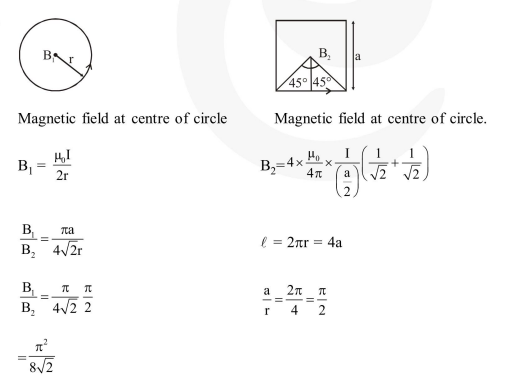Deepak Scored 45->99%ile with Bounce Back Crack Course. You can do it too!

# Two identical wires

Question:

Two identical wires $\mathrm{A}$ and $\mathrm{B}$, each of length ' $T$ ', carry the same current I. Wire $\mathrm{A}$ is bent into a circle of radius $R$ and wire $B$ is bent to form a square of side ' $a$ '. If $B_{A}$ and $B_{B}$ are the values of magnetic field at the centres of the circle and square respectively, then the ratio $\frac{B_{A}}{B_{B}}$ is :

1. $\frac{\pi^{2}}{8 \sqrt{2}}$

2. $\frac{\pi^{2}}{8}$

3. $\frac{\pi^{2}}{16 \sqrt{2}}$

4. $\frac{\pi^{2}}{16}$

Correct Option: 1

Solution: